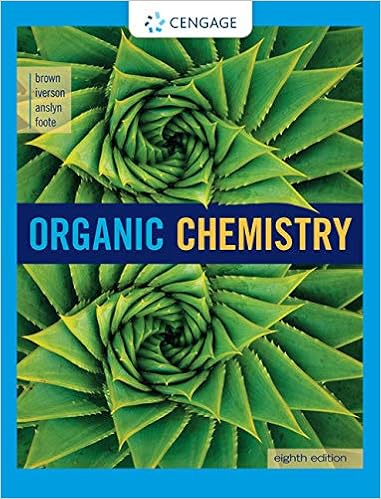# A balance the following reaction h 2 o 2 mno 4 mn 2 o

• Test Prep
• 15
• 100% (1) 1 out of 1 people found this document helpful

This preview shows page 9 - 11 out of 15 pages.

##### We have textbook solutions for you!
The document you are viewing contains questions related to this textbook.The document you are viewing contains questions related to this textbook.
Chapter 2 / Exercise 2.33
Organic Chemistry
BrownExpert Verified
(a) Balance the following reaction.
2
2O}
2O
3
3
+ e
} x 2
+ 2OH
5Cl
(10)Consider the following Molecular Orbitals for a diatomic molecule, those depicted here: σ2p σ2pπ2pπ2pAssign the correct label;π2p, σ2p, π*2porσ*2pto the four (4) pictures on the right (those placed vertically).
##### We have textbook solutions for you!
The document you are viewing contains questions related to this textbook.The document you are viewing contains questions related to this textbook.
Chapter 2 / Exercise 2.33
Organic Chemistry
BrownExpert Verified
9 FINAL EXAM - Karlin page 9 of 14Chem 030.102 / Section 2 Name_ANSWER KEY ______ May 14, 2013 16.(10)Consider the sand three porbitals depicted below. If these are combined, i.e., "hybridized", what kind of hybrid orbitals are formed; what are they called.? How many are there? What is the geometry of molecules formed which use this type of hybridization, i.e., what is the angle formed between atoms that are bonded to some central atom.? What is an example of a real molecule like this?
17.(10)For each of the following ions in their ground-state, predict the type of orbital (1s, 2p, 3d, 4f, etc.) that the electrons of highest energy will occupy. (a) Ti3+(b) In+(c) Te2–(d) 3d Ag+
4d Answers: ________ ________ ________ ________18.(10) (a)Which of the following hydrated cations (i.e., waters are the only ligands) are colorless: Fe2+(aq), Zn2+(aq), Cu1+(aq), Cu2+(aq), V5+(aq), Ca2+(aq), Co2+(aq), Sc3+(aq), Pb2+(aq)? Explain your choice.
V
•••